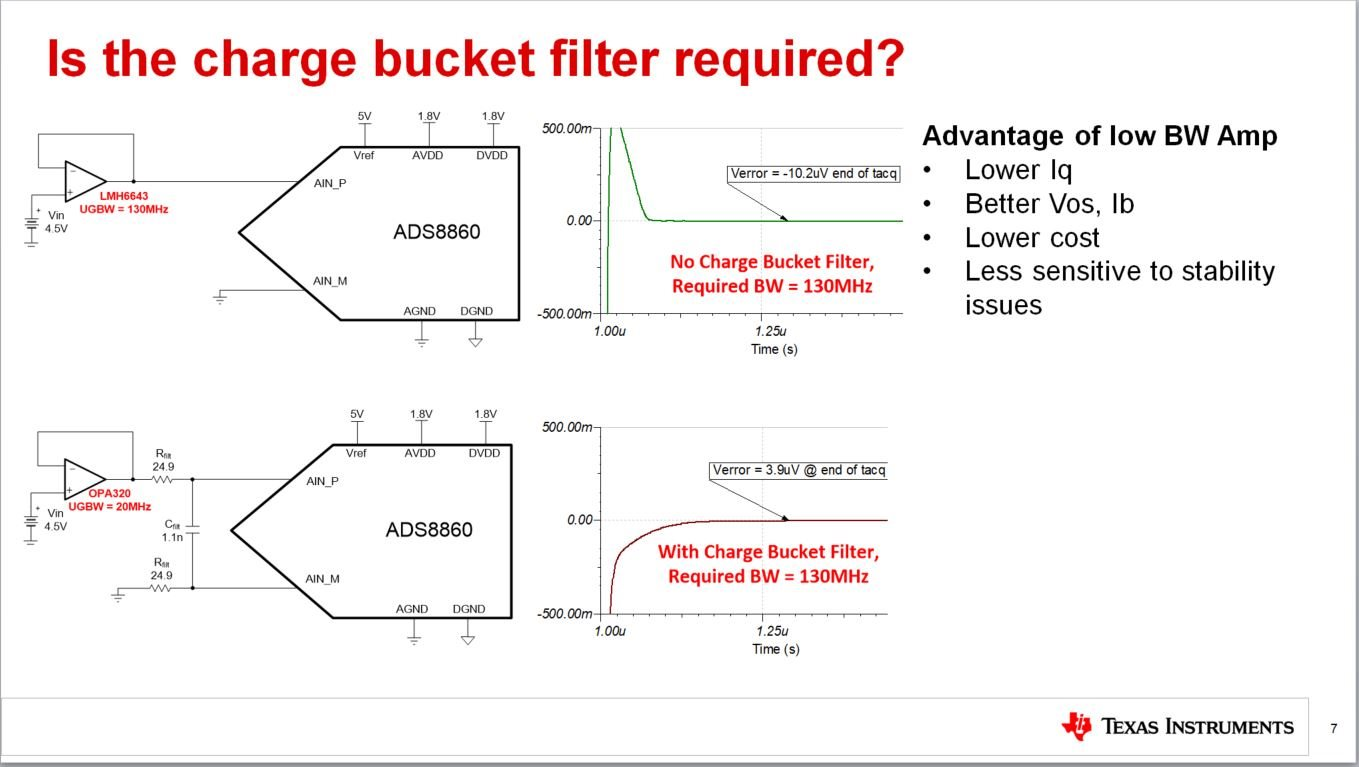If you have a related question, please click the "Ask a related question" button in the top right corner. The newly created question will be automatically linked to this question.

• ResolvedHi, i was studying about charge bucket filter with TI precision LAB.

I kind of can understand why we use Cfilt. By using Cfilt, we can supply enough voltage to Csh.

But in this case, input voltage is DC. In this case, do we also need a High bandwidth OPAMP?

Also, could you explain it in more detail why we can use low bandwidth OPAMP when we use Cfilt when the input is AC?

What is the relationship between OPAMP bandwidth and the speed of capacitor charging?

I think I am little bit confused the concept of DC,AC.

Thanks,

Yun

• Hello Yun,

Regarding your first question, even if the input signal is a DC level, the amplifier output load current is an AC waveform, with a fundamental frequency equal to the input sampling rate of the ADC.  An ideal amplifier has 0 output impedance.  In this case, the load current waveform would not matter, and a very low speed amplifier could be used.  However, all amplifiers have an output impedance.  When the input sampling capacitor of the ADC is switched onto the amplifier output, the sampling capacitor causes a large current to flow, which interacts with the amplifier output impedance resulting in a voltage drop. The change in output voltage is seen by the amplifier inputs through the feedback resistor network, and the amplifier will then respond to force the output voltage back to the desired voltage level.  The time required for this recovery is dependent on the amplifier bandwidth.

By adding a charge bucket capacitor, it provides most of the initial charge required by the input sampling capacitor.  Since the charge bucket capacitance is much larger than the sampling capacitor, the voltage drop is much less verses driving the ADC input without the charge bucket capacitor.  Although the amplifier still must recover the voltage on the charge bucket capacitor, it is a much smaller voltage deviation, allowing a lower bandwidth device to be used.

Regards,
Keith Nicholas